# Review of Statistics Standardization Central Limit Theorem Criteria

• Slides: 25Review of Statistics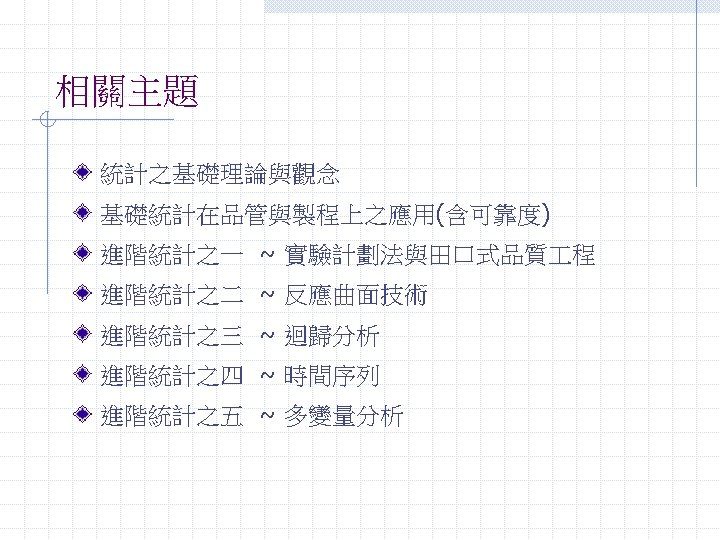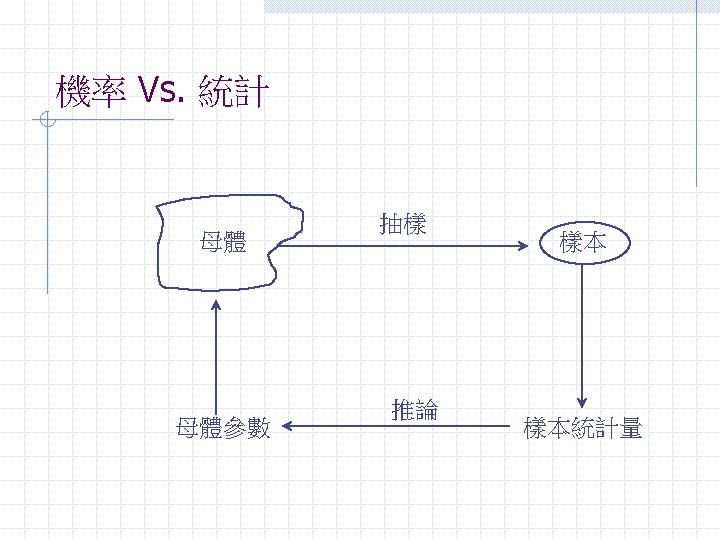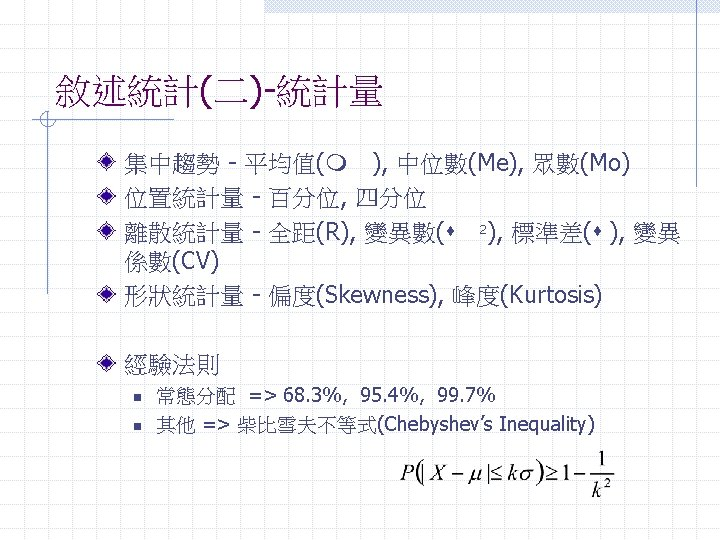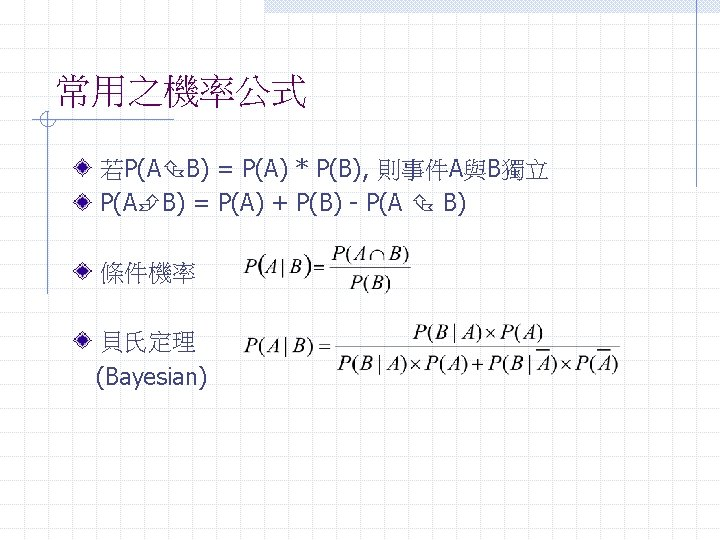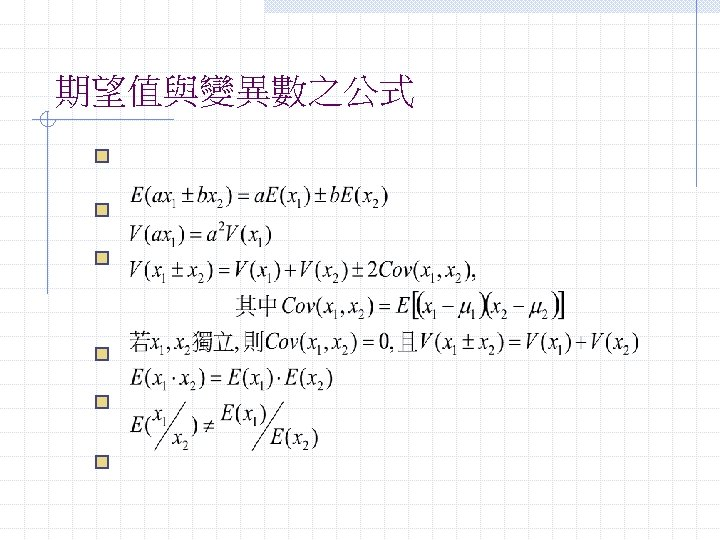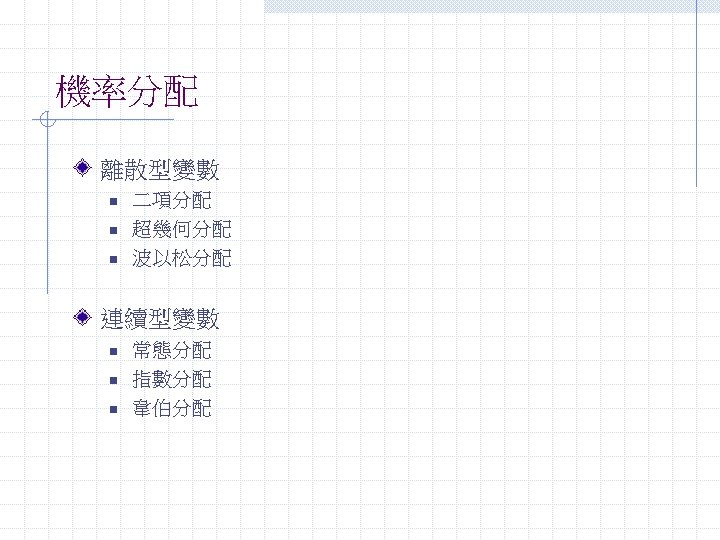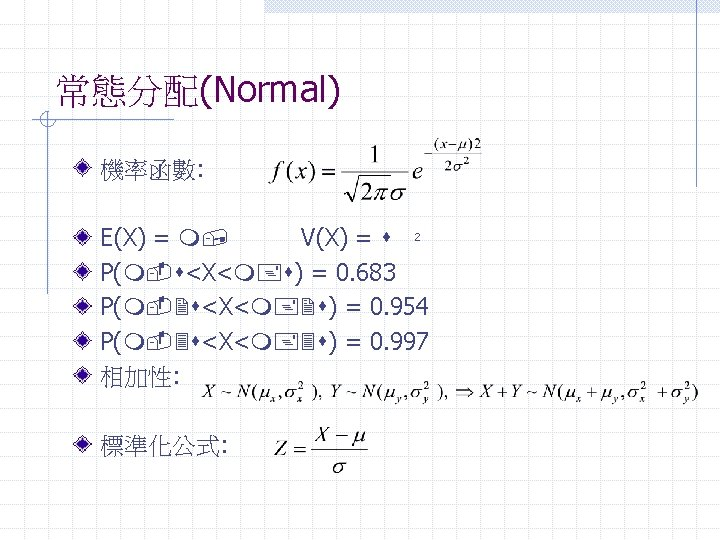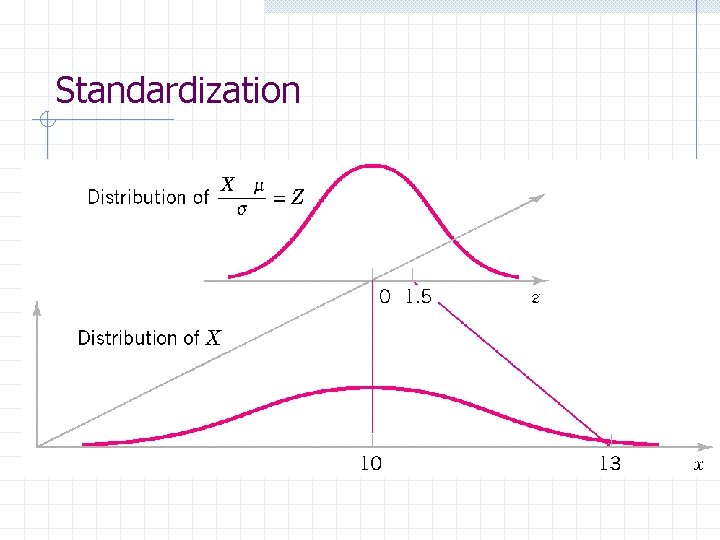StandardizationCentral Limit Theorem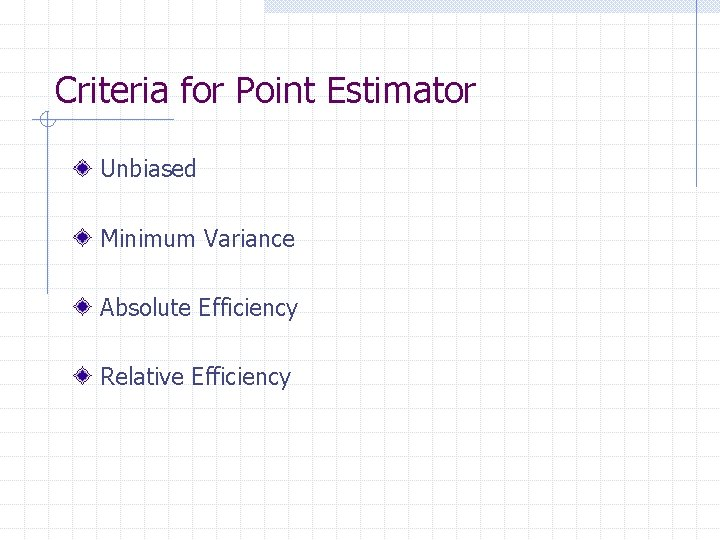Criteria for Point Estimator Unbiased Minimum Variance Absolute Efficiency Relative Efficiency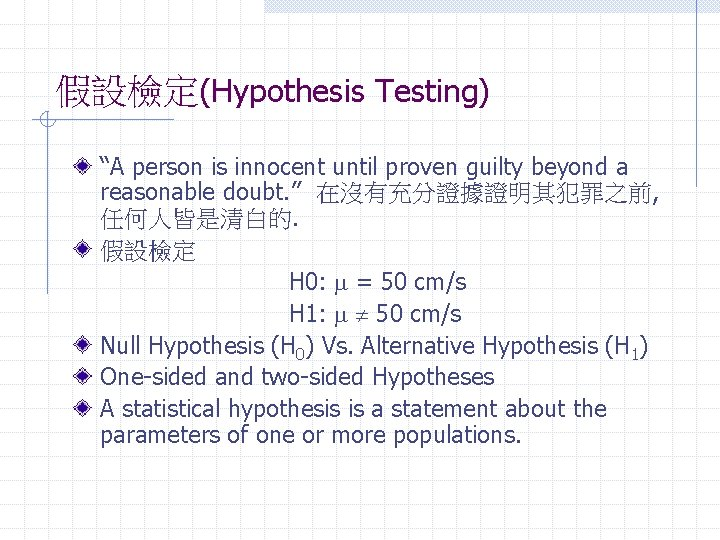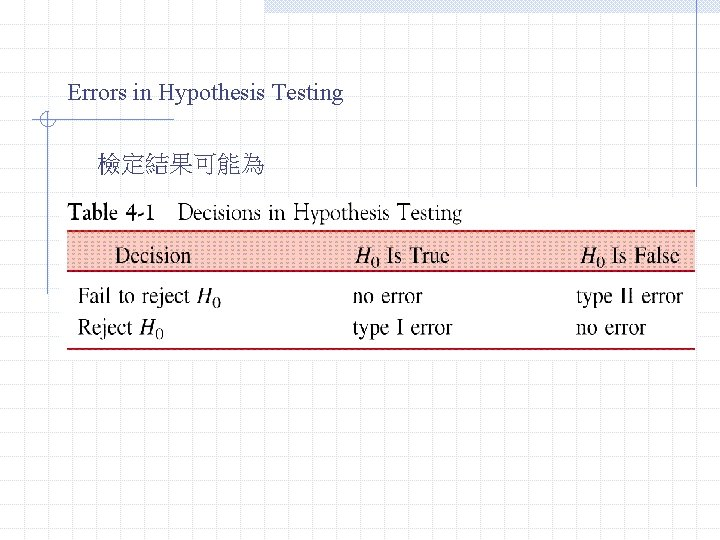Errors in Hypothesis Testing 檢定結果可能為 Type I Error(a): Reject H 0 while H 0 is true. Type II Error(b): Fail to reject H 0 while H 0 is false.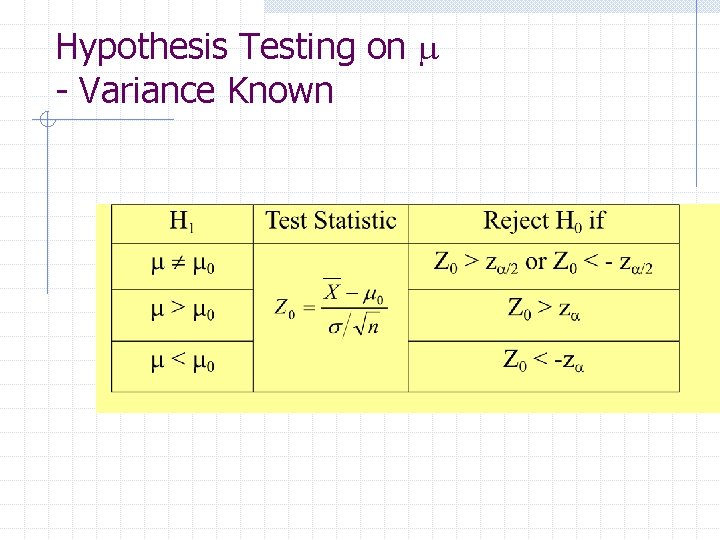Hypothesis Testing on m - Variance KnownConstruction of the C. I. From Central Limit Theory, Use standardization and the properties of Z,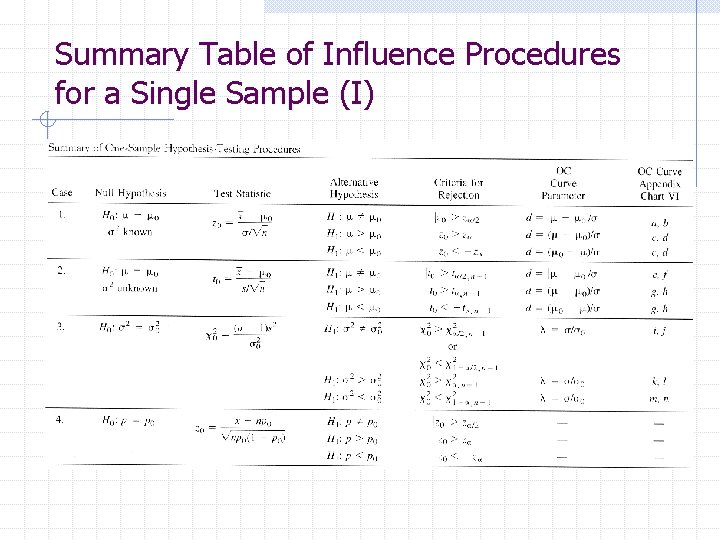Summary Table of Influence Procedures for a Single Sample (I)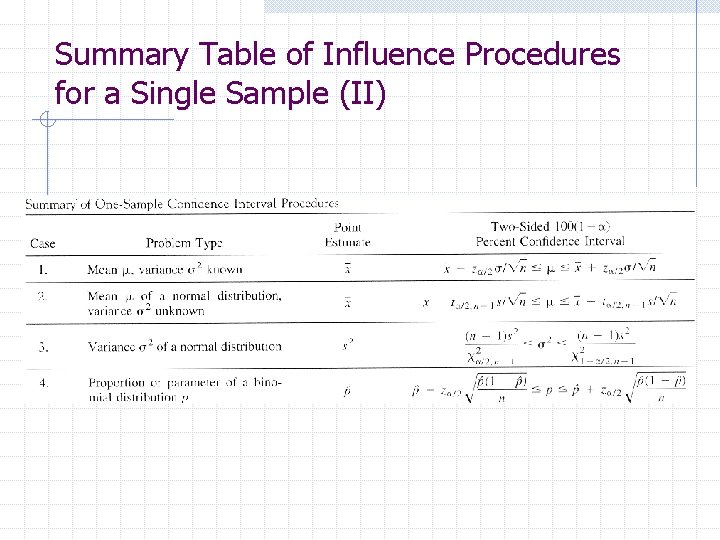Summary Table of Influence Procedures for a Single Sample (II)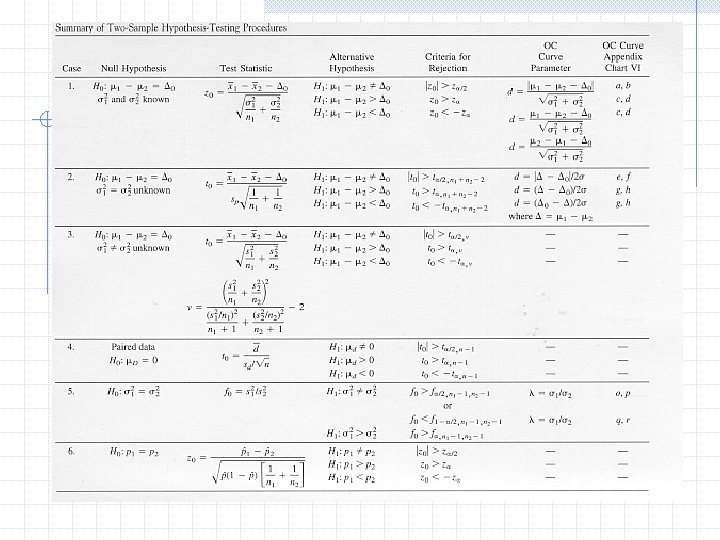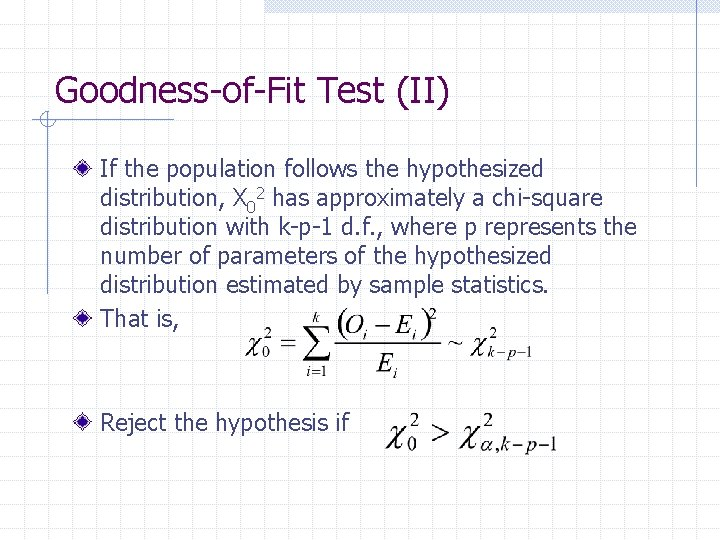Goodness-of-Fit Test (II) If the population follows the hypothesized distribution, X 02 has approximately a chi-square distribution with k-p-1 d. f. , where p represents the number of parameters of the hypothesized distribution estimated by sample statistics. That is, Reject the hypothesis if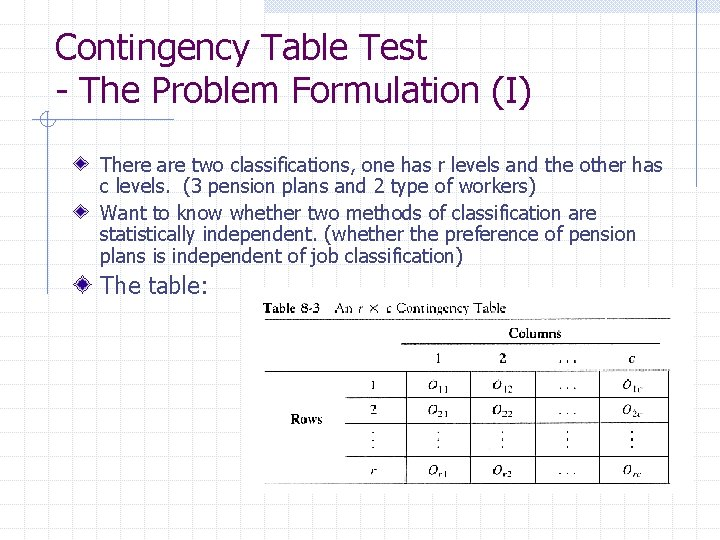Contingency Table Test - The Problem Formulation (I) There are two classifications, one has r levels and the other has c levels. (3 pension plans and 2 type of workers) Want to know whether two methods of classification are statistically independent. (whether the preference of pension plans is independent of job classification) The table: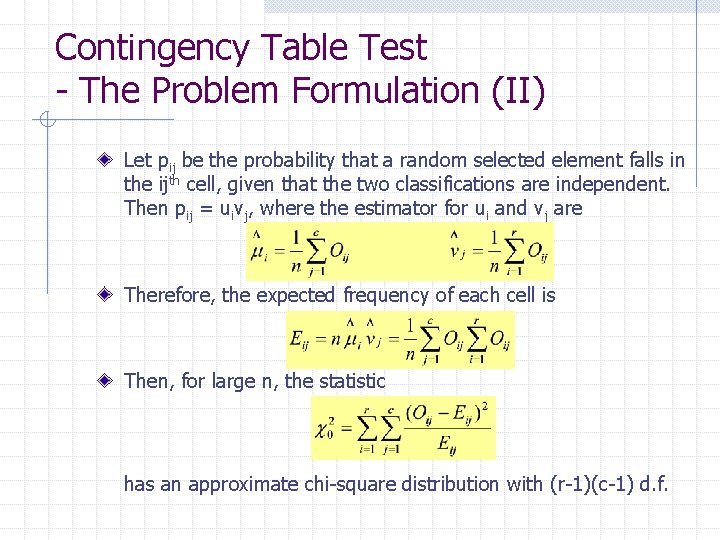Contingency Table Test - The Problem Formulation (II) Let pij be the probability that a random selected element falls in the ijth cell, given that the two classifications are independent. Then pij = uivj, where the estimator for ui and vj are Therefore, the expected frequency of each cell is Then, for large n, the statistic has an approximate chi-square distribution with (r-1)(c-1) d. f.